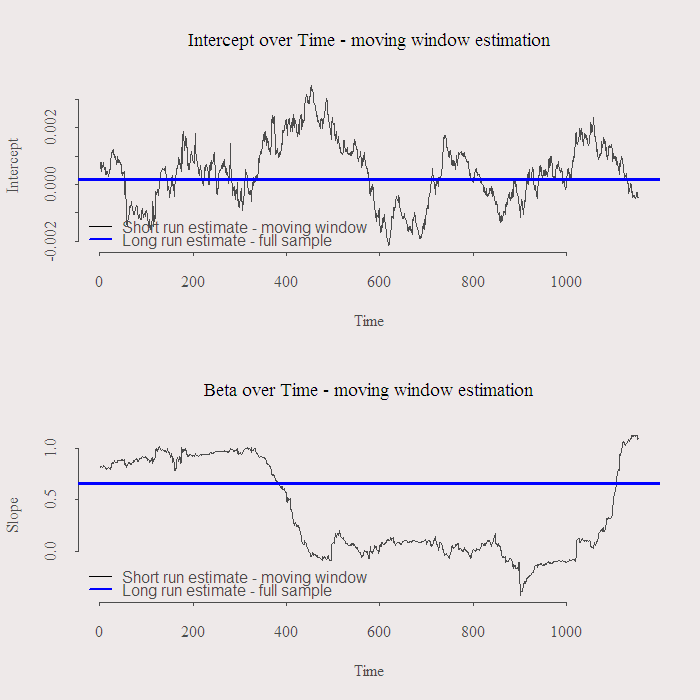# A shrinkage estimator for beta

In the post pairs trading issues one of the problems raised was the unstable estimates of the stock’s beta with respect to the market. Here is a suggestion for a possible solution, which is not really a solution but more stuff to do to make you feel less stupid when trading based on your fragile estimates.
Have a look at the following figure:Rolling coefficients for Microsoft (Regression: MSFT~SPY) - Window of 120 days, Solid blue is beta estimated using the full sample
We can see the intercept is not fluctuating much meaning indeed that if the market does not move, neither does MSFT. The beta however is comfortably fluctuating between a solid market follower, (beta = 1) to neutral (beta = 0), and can be even used to hedge the market at some short period (beta < 0), yeah sure, loads of economic sense in that estimate. Things of course get more volatile with a shorter window, 120 days roughly means the most recent 6 months which is not that short. Maybe we can find a compromise between the long term (stable) estimate and the short term one which might be more relevant but is also more erratic. A way to proceed is to simply average the two estimate. Less simple is to average them in a fancy way using what is commonly known as the shrinkage estimator*. I will shortly provide the figure and code but for now, a flat explanation for this method is that the averaging accounts for the dispersion in the X matrix, in our case it is just the market returns and the intercept, is the current period volatile or not? a more thorough explanation can be given using Singular Value Decomposition of the X matrix but we drop it here. We get a new beta estimate which is an average of the short and long term estimates. We need to decide how much shrinkage to apply. We have a parameter, called hyper parameter since it is chosen rather than estimated from the data, that determines the amount of shrinkage to apply, a low number would mean smaller pull towards the long term estimate and a high number would mean a larger pull towards the long term, so less weight is given to the short term estimate**. The result is:You can see that the more shrinkage you apply the closer the estimate to its long run value. taking the hyper parameter to 0.1 will prevent the beta to fluctuate into negative territory yet still allowing some room for a possible structural changes. You can use this solution to reconcile a volatile estimation procedure with common sense arguments, e.g. might be that beta is indeed negative for this period in time, but does this make sense? might be that your estimate went negative only since you wanted to allow for a structural change, which is a good thing, and this resulted in “not so intuitive” estimate. Enforcing some structure in a smart way might be a simple way to move on. As always, code is given below, thanks for reading.

The following code includes a function to plot your own figures taking as input the time frame, window length and ticker you wish to view.

NOTES:
* The idea is related to “Ridge Regression” and can also be viewed as a semi-bayesian approach where the prior has mean equal to the long term estimate.
– I skip the “interesting” explanation through the Singular Value Decomposition of the predictor matrix.
– The new estimate is biased but whatever, we are not into inference anyway.

** thats specifically for the code, but you may find it is reveresed in other texts, depending on math definitions.

*** “<<-" is a nice operator mentioned in "The art of R programming" book, it writes the object into the upper level.

### 2 comments on “A shrinkage estimator for beta”

1.André de Boer says:

Hi Eran,
The line: wl = 120 ; n = NROW(ret), is not working because ret is not set.
By the way I like your blog.
Regards, André

1.Eran says:

Hi Andre,
Thanks for the comment.
The super-assignment operator writes the variable after you run the function. So plotbet() needs to come first, I updated the post.